# 一、for语句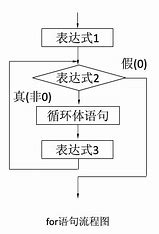for 变量名 in 取值列表
do
命令序列
done

###### 示例：计算从1到100所有整数的和
#!/bin/bash
sum=0
for ((i=1;i<=100;i++ ))
do
sum=$(($sum + $i)) done echo "1-100以内的整数和为：$sum"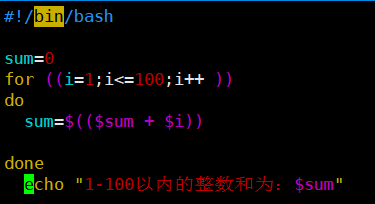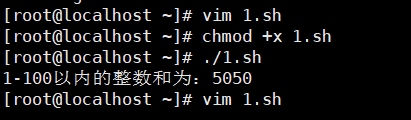###### 示例：过滤出从1到100所有整数的奇偶数
#!/bin/bash
echo -n  "偶数 "
for ((i=1;i<=100;i++))
do
a=$[$i%2 ]
if [ $a = 0 ] then echo -n "$i,"
fi
done
echo -n  "奇数 "
for ((i=1;i<=100;i++))
do
a=$[$i%2 ]
if [ $a = 1 ] then echo -n "$i,"
fi
done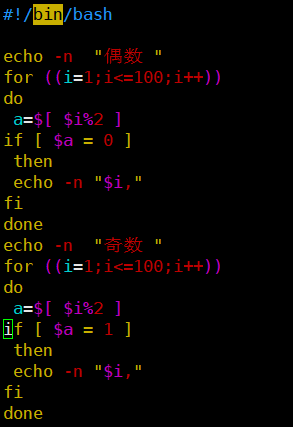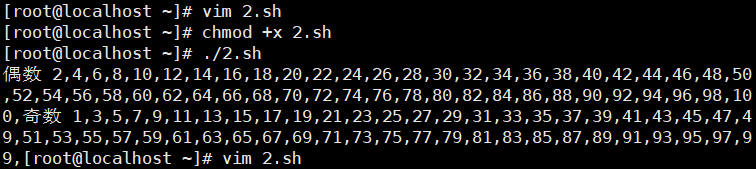###### 示例：提示用户输入一个小于100的整数，并计算从1到该数之间所有整数的和
#!/bin/bash

sum=0
if [ $a -lt 100 ]&>/dev/null then for((i=1;i<=$a;i++))
do
sum=$(($sum+$i)) done echo "1到$n的所有整数和为$sum" elif [$a -ge 100 ]&>/dev/null
then
echo "错误，输入的整数大于100"
else
echo "请正确输入整数"
fi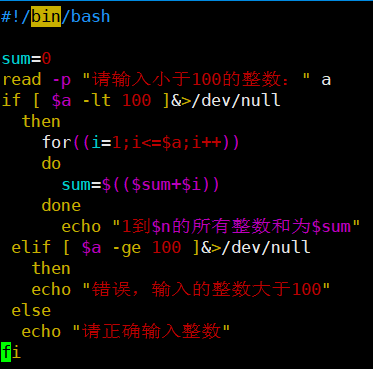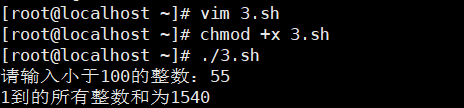###### 示例：求从1到100所有整数的偶数和、奇数和
#!/bin/bash

sum1=0
sum2=0
for((i=1;i<=100;i++))
do
if [ $( expr$i % 2 ) -eq 0 ]
then
sum2=$(($sum2+$i)) else sum1=$(($sum1+$i))
fi

done

echo "100以内的奇数和为：$sum1" echo "100以内的偶数和为：$sum2"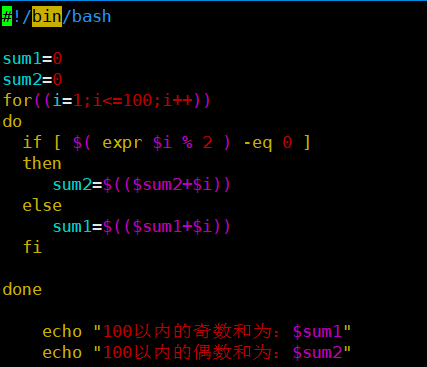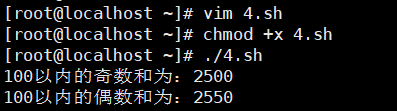# 二、while语句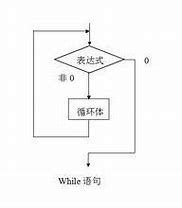while 条件测试操作
do
命令序列
done

###### 示例：计算从1到100所有整数的和
#!/bin/bash

sum=0
i=0
while
[ $sum -lt 100 ] do let sum++ i=$[ $sum +$i ]
done
echo $i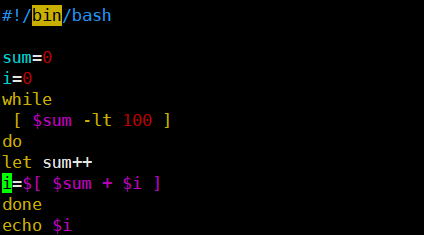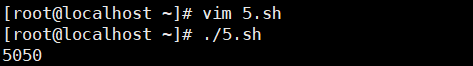###### 示例：过滤出从1到100所有整数的奇偶数 #!/bin/bash i=1 while [$i -lt 100 ]
do
a=$[$i % 2 ]
if [ $a -eq 1 ] then echo -e "奇数为：" "$i\t"
fi

let i++
done

b=1
while [ $b -le 100 ] do c=$[ $b % 2 ] if [$c -eq 0 ]
then
let i++

done

echo $sum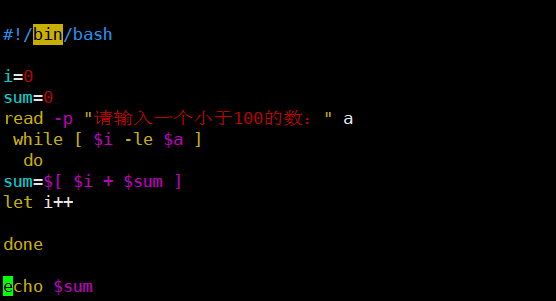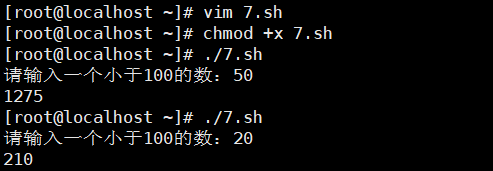###### 示例：求从1到100所有整数的偶数和、奇数和 #!/bin/bash i=1 sum1=0 sum2=0 while [$i -le 100 ]
do

a=$[$i % 2 ]
if [ $a -eq 1 ] then sum1=$[ $sum1 +$i ]
else
sum2=$[$sum2 + $i ] fi let i++ done echo "奇数和为："$sum1
echo "偶数和为：" $sum2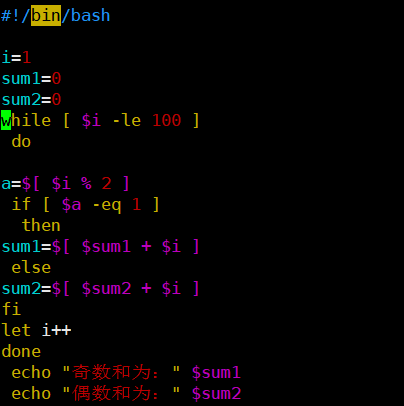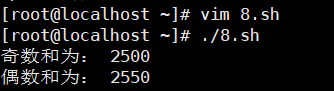# 三、until语法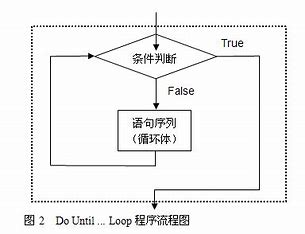until 条件测试操作 do 命令序列 done  ###### 示例：计算1-50的总和 #!/bin/bash a=0 sum=0 until (($a>50))
do
sum=$[$sum + $a ] let a++ done echo$sum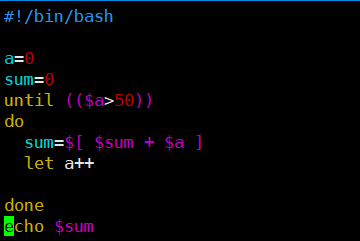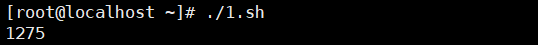# 四、结束循环——break和continue

## 1、break

#!/bin/bash

for ((a=1; a<=5; a++))
do
echo "外循环第$a次" if [$a -eq 3 ]
then
break
fi
for ((b=1; b<=5; b++))
do
echo "内循环第$b次" if [$b -eq 3 ]
then
break
fi
done
done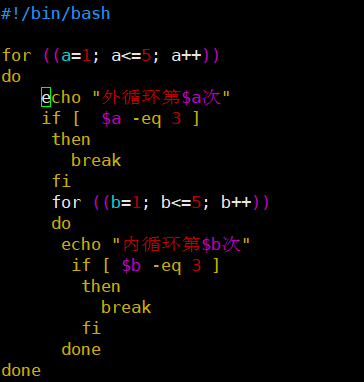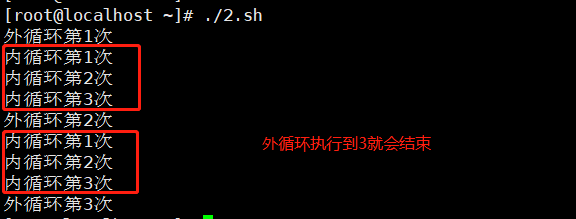## 2、continue

continue :中止某次循环中的命令，但不会完全中止整个命令

#!/bin/bash

for ((a=1; a<=15; a++))
do
if [ $a -gt 5 ] && [$a -lt 10 ]
then
continue
fi
echo "current value: $a" done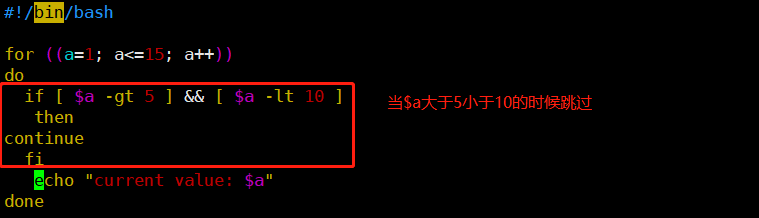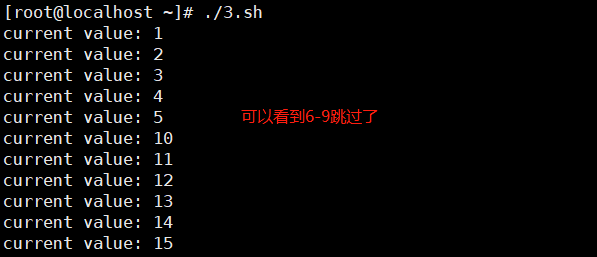# 五、转义字符 echo -n 表示不换行输出 echo -e 输出转义字符，将转义后的内容输出到屏幕上 常用的转义字符如下： \b 转义后相当于按退格键（backspace），但前提是"\b"后面存在字符；"\b"表示删除前一个字符，"\b\b"表示删除前两个字符。 \c 不换行输出，在"\c"后面不存在字符的情况下，作用相当于 echo -n； 但是当"\c"后面仍然存在字符时，"\c"后面的字符将不会被输出。 \n 换行，被输出的字符从"\n"处开始另起一行。 \f 换行，但是换行后的新行的开头位置连接着上一行的行尾 \v 与\f相同 \t 转以后表示插入tab，即横向制表符 \r 光标移至行首，但不换行，相当于使用"\r"以后的字符覆盖"\r"之前同等长度的字符；但是当"\r"后面不存在任何字符时，"\r"前面的字符不会被覆盖 \\ 表示插入"\"本身  # 六、IFS字段分隔符 默认包含 空格，制表符，换行符 查看命令：set | grep IFS IFS=$' \t\n'

IFS=$'\n' IFS=: IFS=',' IFS.OLD=$IFS
IFS=$'\n' ... IFS=$IFS.OLD


12-20350
05-11510
05-13135
05-1672
03-1038
12-21210
12-22180
03-062480
10-1827万+
04-265987
02-2490
05-11117
05-17298
12-211018
10-3122万+
12-20376

### “相关推荐”对你有帮助么？

•非常没帮助
•没帮助
•一般
•有帮助
•非常有帮助被折叠的  条评论 为什么被折叠?到【灌水乐园】发言¥2 ¥4 ¥6 ¥10 ¥20余额支付 (余额：-- )扫码支付获取中扫码支付点击重新获取扫码支付1.余额是钱包充值的虚拟货币，按照1:1的比例进行支付金额的抵扣。
2.余额无法直接购买下载，可以购买VIP、C币套餐、付费专栏及课程。余额充值